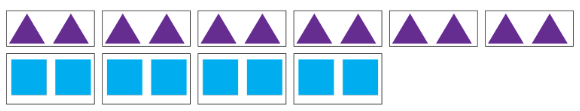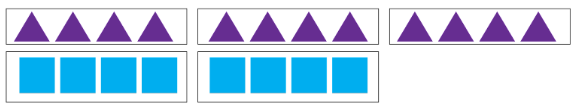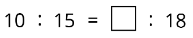Study P5 Mathematics Ratio: Introduction - Geniebook# Ratio: Introduction

In the following article, we will study the topic - Ratio.

The learning objectives are:

1. Idea of ratio
2. Equivalent ratio and its simplest form
3. Ratio of three quantities
4. Word problems involving ratio

## 1. Idea Of Ratio

Ratio is the relationship between two or more items.

It may not represent the actual quantities.

No units are included in ratios.

Look at the diagram below.

There are $$4$$ brown horses and $$1$$ white horse.1. The ratio of the number of brown horses to the number of white horses is $$4 : 1$$.

We read the ratio as $$4$$ is to $$1$$. The sign “:” is read as ‘is to’.

Note: The order is very important. Let’s take a look!

1. The ratio of the number of white horses to the number of brown horses is $$1 : 4$$.
2. The ratio of the number of brown horses to the total number of horses is $$4 : 5.$$

How do we get $$4 : 5$$?

We have $$4$$ brown horses and a total of $$5$$ horses.

Question 1:

Look at the diagram below.What is the ratio of the number of triangles to the number of circles?

1. $$4 : 5$$
2. $$5 : 4$$
3. $$4 : 9$$
4. $$9 : 4$$

Solution:

There are $$5$$ triangles and $$4$$ circles.

The ratio of the number of triangles to the number of circles is $$5 : 4$$

(2) $$5 : 4$$

Question 2:

The table below shows the amount of money each person has.

 Name Amount Of Money Alice Ben Chole Dave $8$5 $7$11

What is the ratio of the amount of money Alice has to the amount of money Chole has?

1. $$8 : 5$$
2. $$8 : 7$$
3. $$7 : 8$$
4. $$8 : 11$$

Solution:

Alice has $$8$$ and Chloe has $$7$$

The ratio of the amount of money Alice has to the amount of money Chloe has is $$8 : 7$$

(2) $$8 : 7$$

Note: We do not include units in the ratio.

## 2. Equivalent Ratios And Its Simplest Form1. The ratio of the number of triangles to the number of squares is $$12 : 8$$.
2. If we group the triangles and squares into groups of $$2$$, the ratio of the number of triangles to the number of squares is $$6 : 4$$.We can get the ratio $$6 : 4$$ by dividing the initial ratio by $$2$$.1. If we place the triangles and squares into groups of $$4$$, then the ratio of the number of triangles to the number of squares is $$3 : 2$$.We can get the ratio $$3 : 2$$ by dividing the initial ratio by $$4$$.1. $$12 : 8, 6 : 4,$$ and $$3 : 2,$$ are equivalent ratios.

$$3 : 2$$ is the simplest form of $$12 : 8$$.

Question 1:

There are $$4$$ squares and $$8$$ circles. Which of the following is not an equivalent ratio of the number of squares to the number of circles?

1. $$1 : 2$$
2. $$1 : 4$$
3. $$2 : 4$$
4. $$4 : 8$$

Solution:(2) $$1 : 4$$

Question 2:

John is $$12$$ years old and May is $$16$$ years old now. What will be the ratio of John’s age to May’s age in $$6$$ years' time? Express the ratio in its simplest form.

1. $$3 : 4$$
2. $$4 : 3$$
3. $$9 : 11$$
4. $$11 : 9$$

Solution:

In $$6$$ years’ time,

John’s age \begin{align*}​\\ &= 12 + 6 \\ &= 18 \end{align*}

May’s age \begin{align*}​\\ &= 16 + 6 \\ &= 22 \end{align*}(3) $$9 : 11$$

Question 3:

Find the missing number1. $$9$$
2. $$12$$
3. $$15$$
4. $$16$$

Solution:(2) 12

## 3. Ratio Of Three Quantities

Question 1:

There are $$4$$ blue marbles, $$6$$ red marbles and $$12$$ green marbles.

1. What is the ratio of the number of red marbles to the number of blue marbles to the number of green marbles in its simplest form?

Solution:$$3 : 2 : 6$$

Question 2:

The table below shows the number of stamps collected by $$4$$ children.

 Name Number Of Stamps Ali Bala Charles David 9 8 15 12

Find the ratio of the number of stamps Bala has to the number of stamps David has to the total number of stamps in its simplest form.

1. $$8 : 12 : 24$$
2. $$2 : 4 : 11$$
3. $$2 : 3 : 11$$
4. $$2 : 3 : 12$$

Solution:

Total number of stamps \begin{align*}​\\ &= 9 + 8 + 15 + 12 \\ &= 44 \end{align*}(3) $$2 : 3 : 11$$

## 4. Word Problems involving Ratio

Question 1:

Janice and Amy shared some sweets in the ratio $$4 : 7$$. Amy received $$9$$ more sweets than Janice. How many sweets did they have altogether?Janice had $$4$$ units and Amy had $$7$$ units.

Difference in number of units between Janice and Amy \begin{align*}​\\ &= 7 \text{ units} - 4 \text{ units}\\ &= 3 \text{ units} \end{align*}

\begin{align*} 3 \text{ units} &= 9 \\ 1 \text{ units} &= 9 \div 3 \\ &= 3 \end{align*}

Total number of sweets Janice and Amy had

\begin{align*}​ &= 4 \text{ units} + 7 \text{ units} \\ &= 11 \text{ units} \\ &= 11 \times 3 \\ &= 33 \end{align*}

$$33$$ sweets

Question 2:

Sandy and Theresa shared $$210$$ in the ratio $$2 : 5$$. How much more did Theresa receive than Sandy?

1. $$30$$
2. $$60$$
3. $$90$$
4. $$150$$

Solution:

\begin{align*} \textbf{Sandy} \quad &\textbf{:} \quad \textbf{Theresa}\\ \textbf{2} \quad &\textbf{:} \quad \textbf{5} \end{align*}

\begin{align*}​ 7 \text{ units} &= 210 \\ 1 \text{ unit} &= 210 ÷ 7 \\ &= 30 \end{align*}

Difference between Theresa and Sandy

\begin{align*}​ &= 5 \text{ units} – 2 \text{ units} \\ &= 3 \text{ units} \\ &= 3 \times 30 \\ &= 90 \end{align*}

(3) $$90$$

Question 3:

Peter has some red and blue marbles in the ratio $$6 : 5$$. He has $$30$$ red marbles. How many marbles does he have altogether?

1. $$25$$
2. $$31$$
3. $$41$$
4. $$55$$

Solution:

\begin{align*} \textbf{Red} \quad &\textbf{:} \quad \textbf{Blue}\\ \textbf{6} \quad &\textbf{:} \quad \textbf{5} \end{align*}

\begin{align*}​ 6 \text{ units} &= 30 \\ 1 \text{ unit} &= 30 \div 6 \\ &= 5 \end{align*}

Total number of marbles Peter has

\begin{align*}​ &= 6 \text{ units} + 5 \text{ units} \\ &= 11 \text{ units} \\ &= 11 \times 5 \\ &= 55 \end{align*}

(4) $$55$$

## Key Points to Remember:

• Ratios are used to compare two or more quantities.
• Ratios may not represent the actual quantities.
• No units are included in ratios.
• Similar to fractions, do express ratios in its simplest form unless stated otherwise.

## Conclusion

In this article, we touched on the idea of ratio. Ratio is a comparison between two or more quantities. We learnt about the equivalent ratios and how to convert them into the simplest form. We also studied the ratio of three quantities and solved some word problems involving ratios.

Continue Learning
Volume Of A Liquid Decimals - Operations & Conversions
Ratio: Introduction Average - Formula
Percentage, Fractions And Decimals Whole Numbers
Strategy - Equal Stage Angle Properties
Table Rates Whole Number Strategy: Gap & Difference
Fractions - Addition & Subtraction Ratio Strategy: Repeated IdentityPrimaryPrimary 1Primary 2Primary 3Primary 4Primary 5EnglishMaths
Volume Of A Liquid
Decimals - Operations & Conversions
Ratio: Introduction
Average - Formula
Percentage, Fractions And Decimals
Whole Numbers
Strategy - Equal Stage
Angle Properties
Table Rates
Whole Number Strategy: Gap & Difference
Ratio Strategy: Repeated IdentitySciencePrimary 6SecondarySecondary 1Secondary 2Secondary 3Secondary 4